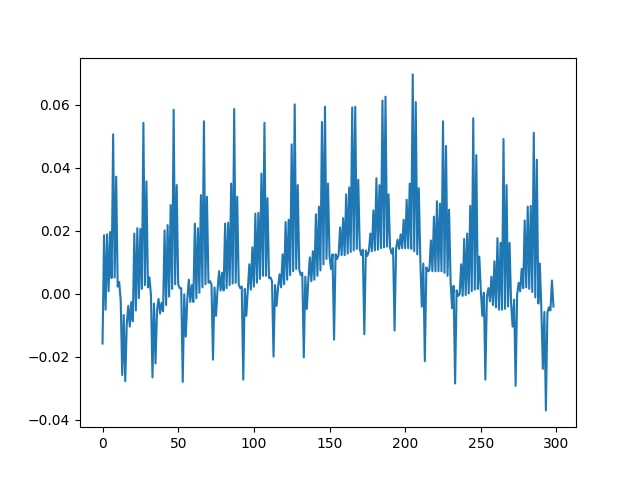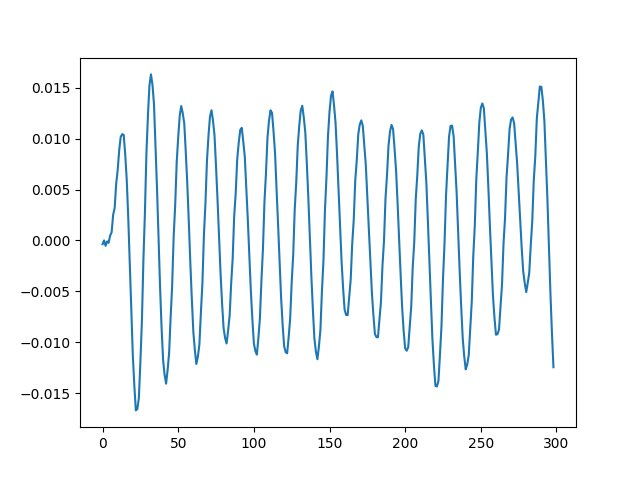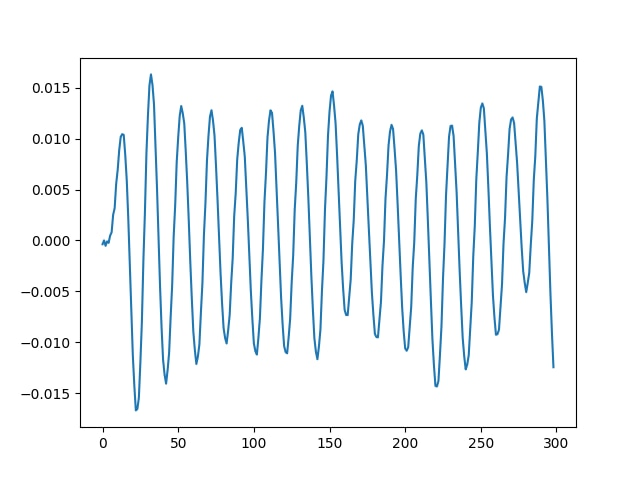## Overview

This guide provides a simple example of how to use the CMSIS-DSP Python wrapper and how a CMSIS-DSP API is represented in Python.

Signal processing algorithms on embedded systems are often implemented with a library of optimized functions using a reference developed in a scientific computing environment. Popular environments include the open source Python library, SciPy, or MathWorks MATLAB software.

There are always differences between a scientific environment and a library of optimized functions.

Those differences may exist for different reasons, depending on:

• What conventions are used, for example different normalization factors
• What memory layout is used for the parameters of functions, for example the order of filter coefficients
• Whether fixed-point arithmetic is used in the embedded system and floating-point arithmetic is used in the reference environment

These differences mean that translating the theoretical signal processing chain from the scientific environment to the embedded system does not happen immediately. The differences must be understood and under control.

To help, the Arm Digital Signal Processing (DSP) software team has introduced a Python wrapper for the CMSIS-DSP library that is compatible with NumPy. The CMSIS-DSP library is a rich collection of DSP functions that Arm has optimized for the various Arm Cortex-M processors. CMSIS-DSP is widely used in the industry, and also enables optimized C code generation from various third-party tools.

With this Python wrapper, you can design your signal processing algorithm with SciPy and progressively replace part of it with CMSIS-DSP functions that are callable from Python. Once your algorithm has been validated with the CMSIS-DSP functions, you can port the algorithm to your embedded system. This is because the Python API follows the C API of CMSIS-DSP as closely as possible.

The wrapper makes it easy to experiment and ensure that your final implementation will behave as intended.

This guide will show how to use the CMSIS-DSP Python API. At the end of this guide you'll be able to use the CMSIS-DSP Biquad filter to filter a signal defined in Python, plot the result and compare with the SciPy version.

The source code for this guide can be found in the CMSIS-DSP Python Wrapper

This guide will explain the steps related to CMSIS-DSP only. The example.py is contains Python code to load the data and build the SciPy filter.

The ECG data was taken from the PhysioNet database:

Goldberger AL, Amaral LAN, Glass L, Hausdorff JM, Ivanov PCh, Mark RG, Mietus JE, Moody GB, Peng C-K, Stanley HE. PhysioBank, PhysioToolkit, and PhysioNet: Components of a New Research Resource for Complex Physiologic Signals. Circulation 101(23):e215-e220 [Circulation Electronic Pages; http://circ.ahajournals.org/cgi/content/full/101/23/e215]; 2000 (June 13).

And was created for the master thesis:

Lugovaya T.S. Biometric human identification based on electrocardiogram. [Master's thesis] Faculty of Computing Technologies and Informatics, Electrotechnical University "LETI", Saint-Petersburg, Russian Federation; June 2005.

## Before you begin

In order to complete this guide, you need to be familiar with CMSIS-DSP and Python. Our blog Signal processing capabilities of Cortex-M devices provides some useful background information.

Before you begin, you will need to access the wrapper. You can do this using the Python wrapper folder in the CMSIS-DSP.

The signal used as example in this guide is coming from the PhysioNet database that is mentioned in the Overview.

## How to implement biquads to filter an Electrocardiography signal

In this guide, we will see how to filter an Electrocardiography (ECG) signal using a biquad filter defined with SciPy and by calling the CMSIS-DSP functions from Python. We will check that we get the same results with both methods. The biquad filter will remove the high frequency noise. This guide won't explain how to design such a biquad filter.

You can see a a sample ECG signal in the following image (generated with Python):We want to remove the high frequency noise from the signal.

First, let’s import some libraries that we will need for our example:

```import cmsisdsp as dsp
import numpy as np
from scipy import signal
from pylab import figure, plot, show```

Here is an implementation of the filter in SciPy, which shows three biquad filters in sequence. The biquads are described with their poles and zeros.

```p0 = np.exp(1j*0.05) * 0.98
p1 = np.exp(1j*0.25) * 0.9
p2 = np.exp(1j*0.45) * 0.97

z0 = np.exp(1j*0.02)
z1 = np.exp(1j*0.65)
z2 = np.exp(1j*1.0)
g = 0.02```

With those poles and zeros, the filter can be created with:

```sos = signal.zpk2sos(
[z0,np.conj(z0),z1,np.conj(z1),z2,np.conj(z2)]
,[p0, np.conj(p0),p1, np.conj(p1),p2, np.conj(p2)]
,g)```

Now, we filter the signal with the biquad filter and display the first 300 samples of the result (nb is equal to 300).

```res=signal.sosfilt(sos,sig)
figure()
plot(res[1:nb])```

The high frequency noise has now been removed, as you can see in the following image:Let’s replace this filter with a Q31 implementation using CMSIS-DSP and check that we get the same result at the end.

First, we need to create an instance object. In CMSIS-DSP, an instance object corresponds to a C struct which must be allocated, and which contains the filter coefficients and the filter state. The name of the Python function is the name of the C type in CMSIS-DSP.

` biquadQ31 = dsp.arm_biquad_casd_df1_inst_q31()`

To initialize this instance with the CMSIS-DSP initialization function, we need a state array.

The state array must be big enough. According to the CMSIS-DSP documentation, we need four samples for each biquad. Our example includes three biquad

```numStages=3
state=np.zeros(numStages*4)```

To initialize the instance object, we also need a coefficient array that contains the filter coefficients.

SciPy and CMSIS-DSP do not save the coefficients in the same way in memory. In CMSIS-DSP, the a0 coefficient is assumed to be 1 and is not saved in memory. Also, in CMSIS-DSP the "a" coefficients are negative compared to the SciPy conventions.

So, to use the coefficients with CMSIS-DSP we need to convert them.

Finally, to avoid saturations in the Q31 implementation we need to scale down the coefficients by 4. The following Python lines are doing the conversions:

```coefs=np.reshape(np.hstack((sos[:,:3],-sos[:,4:])),15)
coefs = coefs / 4.0```

And of course, the coefficients need to be converted to Q31 format. The toQ31 is a simple Python function that we wrote to convert the float into an integer using Q31 format.

`coefsQ31 = toQ31(coefs)`

Now that we have our coefficients, we can initialize our filter instance. Since the coefficients were scaled down by 4, we need to set the postshift to 2 in the filter as explained in the CMSIS-DSP documentation.

The API arm_biquad_cascade_df1_init_q31 is a direct copy of the C version and would be used in the same way in the C implementation.

```postshift = 2

Now that the filter instance has been initialized, we are nearly ready to filter our signal. But before testing the filter, we also need to convert the input signal to Q31 format:

`sigQ31=toQ31(sig)`

Now we can filter our Q31 signal using the CMSIS-DSP biquad function. Here we limit the signal to only the first 300 samples (nb is equal to 300).

The API arm_biquad_cascade_df1_q31 is a near copy of the C one. The only difference is that the blockSize parameter is inferred from the signal array to make the API a bit easier to use from Python. Also, the CMSIS-DSP pDst parameter is transformed into a return value, so that the API is easier to use. Apart from these diffferences, the API is a copy of the C API.

`res2=dsp.arm_biquad_cascade_df1_q31(biquadQ31,sigQ31[1:nb])`

The result res2 from the function must be converted back to float before being displayed. This is because res2 is a Q31 signal. Once done, the float version can be displayed to check that the filter is behaving as intended with no saturation issues.

```figure()
plot(res2[1:nb])
show()```

And we see on the picture that we get the intended result:Without this Python API, testing this example would have required much more work, including:

• A C implementation
• Generation of test patterns
• A test framework to load the input test pattern and read the output from the execution environment. It could be a board or simulator.

## Related information

DSP for Cortex-M

• Ask development questions, and find articles and blogs on specific topics from Arm experts at the Arm community.

## Next steps

This guide has explained you how to use the CMSIS-DSP with Biquad as examples. Now you can design your signal processing chain in SciPy and then convert it for use in CMSIS-DSP in Python. Once you get something working, you are ready to write the C version which will be very close to the Python code you have written.

After reading this guide, you are ready to get more details from the README file in the CMSIS-DSP folder.

This README will explain to you how other CMSIS-DSP APIs are represented in Python. For most of them, It is similar to what has been explained in this guide. For a few APIs, like the Matrix ones, there are a few differences to make the API easier to use from Python.

Thank you for reading our guide on using the CMSIS-DSP Python wrapper. We look forward to seeing all the cool stuff you will create with this Python wrapper and the CMSIS-DSP library.

If you have any questions when using the Python wrapper for CMSIS-DSP, please create a GitHub issue.